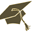Thesis (Selection of subject)(version: 285)
Assignment details
Login via CASPretentious approach to analytic number theory
Thesis title in Czech: Předstírající přístup k analytické teorii čísel Pretentious approach to analytic number theory analytická teorie čísel, předstírající přístup, aritmetická funkce, rozložení prvočísel analytic number theory, pretentious approach, arithmetic function, distribution of prime numbers 2017/2018 diploma thesis angličtina Department of Algebra (32-KA) Mgr. Vítězslav Kala, Ph.D. hidden- assigned and confirmed by the Study Dept. 01.10.2017 01.10.2017 27.11.2017 14.06.2018 09:00 09.05.2018 11.05.2018 14.06.2018 Mgr. Jaroslav Hančl
 Guidelines The recently developed "pretentious approach" to analytic number theory studies the asymptotic behavior of arithmetic functions using Halasz's theorem from 1975. This allows one to prove a number of important results in analytic number theory without relying on the analytic continuation of the Riemann zeta function. The goal of the thesis is to study and compare the classical and pretentious proofs of such theorems, such as the prime number theorem, Siegel-Walfisz theorem or Halasz theorem, and possibly try to further generalize some of these results.
 References A. Granville, A. J. Harper, K. Soundararajan: A more intuitive proof of a sharp version of Halász's theorem, 2017, arXiv:1706.03755 D. Koukoulopoulos: Pretentious multiplicative functions and the prime number theorem for arithmetic progressions, Compos. Math., 149 (2013), no. 7, 1129-1149 D. Koukoulopoulos: The distribution of prime numbers, online lecture notes, http://dms.umontreal.ca/~koukoulo/documents/notes/primes.pdf

Charles University | Information system of Charles University | http://www.cuni.cz/UKEN-329.html